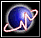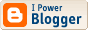Yet another weird SF fan
 I'm a mathematician, a libertarian, and a science-fiction fan. Common sense? What's that?Go to first entry

## Archives

<< current

 Yet another weird SF fan

### What Do Countable Ordinals Look Like?

I've been wondering what countable ordinals look like when graphed as relations. You can think of a countable ordinal α as an order relation >α such that there is a one-to-one order-preserving function f from the natural numbers to the ordinals less than α. In other words, n >α m if and only if f(n) > f(m) for all natural numbers n and m. You can also think of relations on the natural numbers N as subsets of N × N. It's possible to graph the corner of that near the origin.

We start with ω, the supremum of 0, 1, 2, …. In this case, we can use the usual ordering of the natural numbers:As you can see, it is extremely simple.

Next, we look at 2ω, the supremum of ω, ω + 1, ω + 2, …. In this case, we identify the even numbers 2n with the ordinals n and the odd numbers 2n + 1 with the ordinals ω + n:Each half of this has a uniform period.

Then we look at ω2, the supremum of 0, ω, 2ω, …. Here, we use the standard method of constructing a one-to-one correspondence between the natural numbers and ordered pairs of natural numbers. For example, 0 is identified with 0ω + 0; 1 and 2 are identified with 1*ω + 0 and 0*ω + 1, respectively; 3, 4, and 5 are identified with 2*ω + 0, 1*ω + 1, and 0*ω + 2, respectively; etc.:This looks periodic, but the periods expand linearly with the number of periods from the origin.

We continue with ωω, the supremum of ω, ω2, ω3, …. In order to come up with an ordinal corresponding to number n, we first express it in binary. We then start with ordinal 0 and go through the binary expression from the most significant bit to the least adding one at each 1 and multiplying by ω at each 0. For example, 42 has a binary expansion of 101010. It is easy to see this becomes ω3 + ω2 + ω. The graph is:Here, the periods expand exponentially with the number of periods from the origin.

Finally, we look at ε0, the supremum of ω, ωω, ωωω, …. In order to come up with an ordinal corresponding to number n, we take its prime factorization. Multiplying two numbers is equivalent to adding to ordinals. The ordinal corresponding to the nth prime (in which 2 is the first prime, 3 the second, etc.) is ω to the ordinal corresponding to n. Since 1 is the product of no primes, it corresponds to ordinal 0. (As a result, the graph has (1,1) at the origin instead of (0,0).) As an example, let us considers the ordinal corresponding to 42. 2 becomes ω. 3 becomes ωω. 4 becomes ω2. Since 7 is the fourth prime, it becomes ωω2. 42 is 2 * 3 * 7 and the corresponding ordinal is ωω2 + ωω + ω. The graph is:This looks like a humanly-incomprehensible jumble. On the other hand, someone more ingenious than I am might be able to devise something clearer.

I'm not going to touch Γ0 and larger ordinals for now. In any case, ω1CK is too large to be graphed in this manner.

You can find a review of the ordinals displayed above in the chapter “Birthday Cantatatata…” in Gödel, Escher, Bach by Douglas R. Hofstadter.

These ordinals are displayed using JavaScript on my other blog. I would appreciate bug reports from my readers.

Addendum: There was a bug in the original program for ε0. It has been fixed.

 Profiles My Blogger ProfileX-treme Tracker The Atom Feed